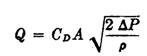﻿ Hydraulic Valve Characteristic – Hydraulic Schematic Troubleshooting

## Hydraulic Valve Characteristic

Proportional valves provide control of fluid power by a combination of variable-area orifices or in some cases by a combination of both fixed and variable orifices. By varying the area of the orifices, the desired relationship of flow and pressure drop through the orifices may be accomplished. The following equation gives this relationship:where Q = flow through the orifice,
CD = coefficient of discharge for the orifice
A = cross-sectional area of the orifice
AP = pressure drop through the orifice
p = mass density of the fluid

The coefficient of discharge CD does not remain a constant but varies depending on the fluid characteristics and the size and configuration of the orifice. The combination of fluid and orifice characteristics may be represented non dimensionally by a curve of discharge coefficient versus Reynolds number (Re). For orifices the Reynolds number is defined by the following equation:

Re = 4mV/p